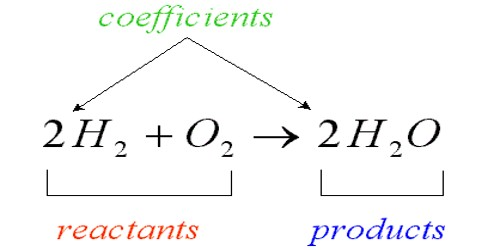Chemistry

# A Chemical EquationA chemical equation is a way to predict the way that two or more chemicals will work together. It shows the chemical formulas of substances that are reacting and the substances that are produced. Using what chemists know about the way chemicals act, we add the letter symbols together just like a math problem. It’s a written representation, using numbers and symbols, of the process that occurs during a chemical reaction. In this way, we can correctly guess if we will get a new chemical when we mix two or more chemicals together, and what that chemical will be.

The elements in an equation are denoted using their symbols. Chemical equations are either worded or written using the elements’ symbols, how much of the element and in what state (solid[s], liquid[l], gas[g]) it is in. A chemical equation is written with the reactants on the left side of an arrow and the products of the chemical reaction on the right.

For example: An aqueous solution of sodium chloride (NaCl[aq]) and another aqueous solution of silver nitrate (AgNO3[aq]). These mixed together form sodium nitrate (NaNO3[aq]) and silver chloride (AgCl[s])

Which in symbols is: NaCl(aq) + AgNO3(aq) → NaNO3(aq) + AgCl(s)

The solutions formed the solid AgCl. This formation can be called a precipitate and the reaction between the two solutions a precipitation reaction, because the solid produced is not dissolved, whereas all the other products are dissolved.